# Calculus 1 : Other Differential Functions

## Example Questions

### Example Question #81 : Other Differential Functions

Find the derivative of the function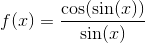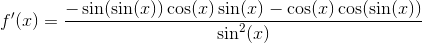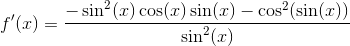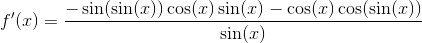Explanation:

To find the derivative of this function we must use the Chain Rule and the Quotient Rule. Applying the Chain Rule to the numerator gives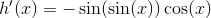Now using the Quotient Rule for the function, we find the derivative to be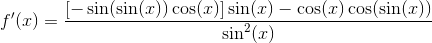### Example Question #81 : Other Differential Functions

Find the derivative of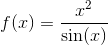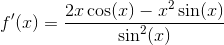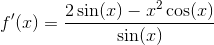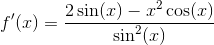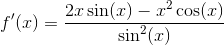Explanation:

To find the derivative of this function, we must use the Quotient Rule which is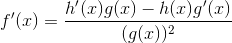Applying this to the function we are given, with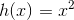and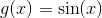gives us### Example Question #81 : How To Find Differential Functions

Find the derivative of the following function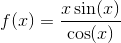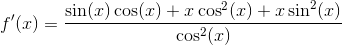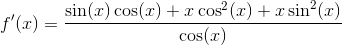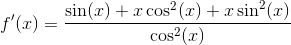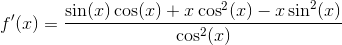Explanation:

To find the derivative of this function we must use the Product Rule and the Quotient Rule. Appling the Product Rule to the numerator of the function gives us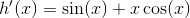Using this with the Quotient Rule, we find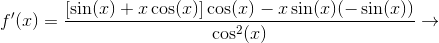### Example Question #84 : How To Find Differential Functions

Find the derivative of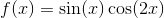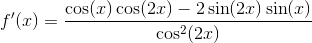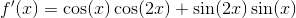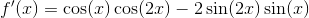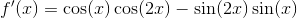Explanation:

To find the derivative of this function we must use the Product Rule and the Chain Rule. If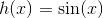and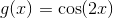then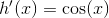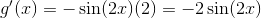Applying these derivatives to the Product Rule gives us### Example Question #85 : How To Find Differential Functions

Differentiate: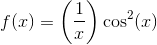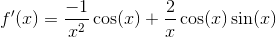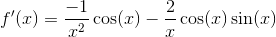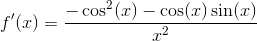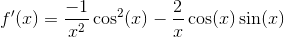Explanation:

To find the derivative of this function we must use the Product Rule and the Chain Rule. First we set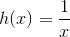and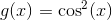Now differentiating both of these functions gives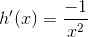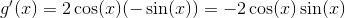Applying this to the Product Rule gives us,### Example Question #86 : How To Find Differential Functions

Find the derivative of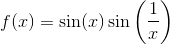None of these answers are correct.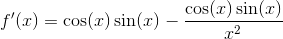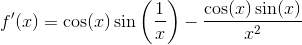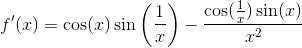Explanation:

To find the derivative of this function we must use the Product Rule and the Chain Rule. If we haveand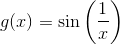thenand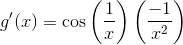Applying this to the product rule, we find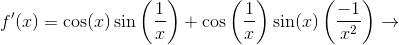### Example Question #87 : How To Find Differential Functions

Find the derivative of the function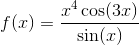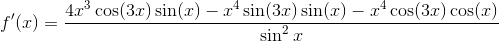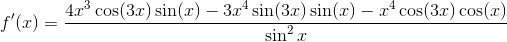None of these answers are correct.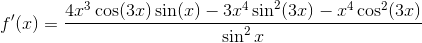Explanation:

To find the derivative of this function, we must use the Product Rule, Quotient Rule, and the Chain Rule. To do this, we first find the derivative of each part.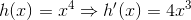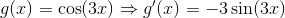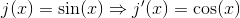Using the derivatives of each part we can find the derivative of the numerator using the Product Rule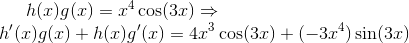Finally, putting this into the Quotient Rule gives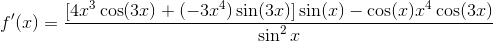### Example Question #88 : How To Find Differential Functions

Differentiate the function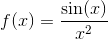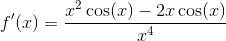None of these answers are correct.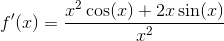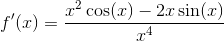Explanation:

To differentiate this function we must use the Quotient RuleUsingand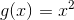.

The derivative of the function is then### Example Question #89 : How To Find Differential Functions

Differentiate the following function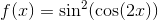None of these answers are correct.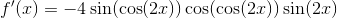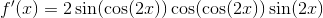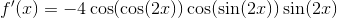Explanation:

To differentiate this function we must use the Chain Rule. where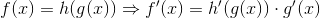Therefore the derivative of the function is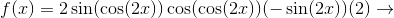### Example Question #81 : Other Differential Functions

Find the derivative of the function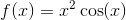None of these answers are correct.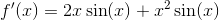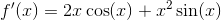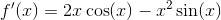Explanation:

To find the derivative of the function, we must use the Product Rule,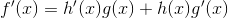Usingand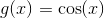.

Therefore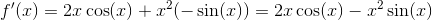### All Calculus 1 Resources